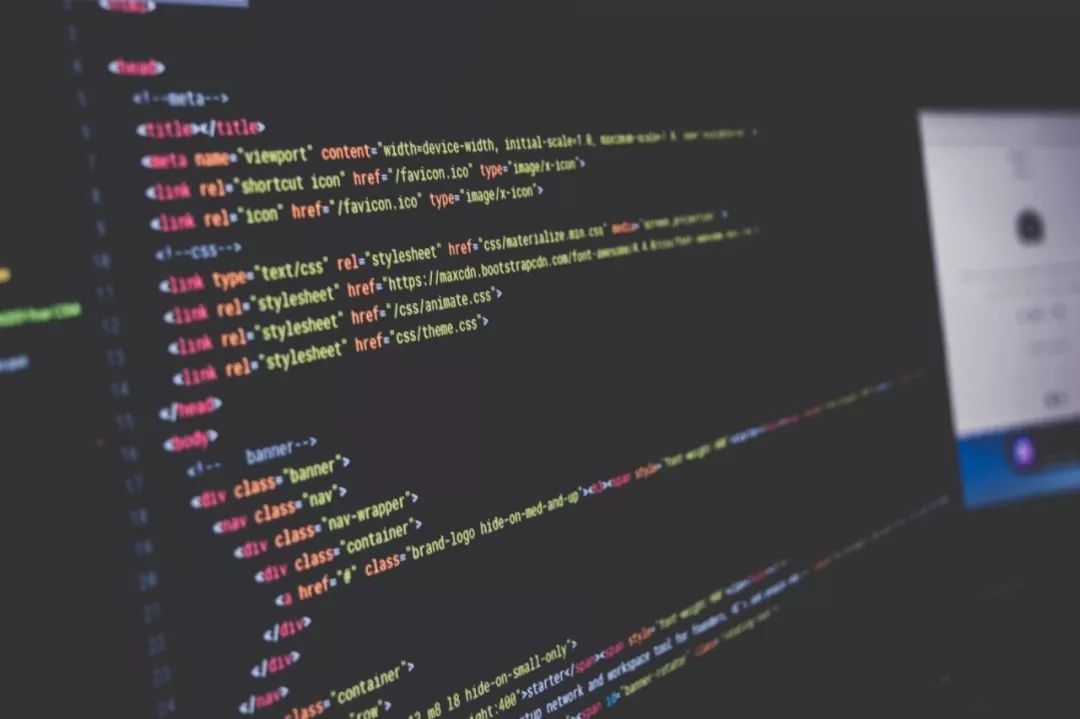2018年4月26日

# 编程快速入门，必备技能！Photo: Sai Kiran Anagani/Unsplash

## 6、写地道的代码

def matrix_of_floats(matrix_of_anything):

n = len(matrix_of_anything)

n_i = len(matrix_of_anything)

new_matrix_of_floats = []

for i in xrange(0, n):

row = []

for j in xrange(0, n_i):

row.append(float(matrix_of_anything[i][j]))

new_matrix_of_floats.append(row)

return new_matrix_of_floats

def matrix_of_floats(matrix_of_anything):

return [[float(a_ij) for a_ij in a_i]

for a_i in matrix_of_anything]